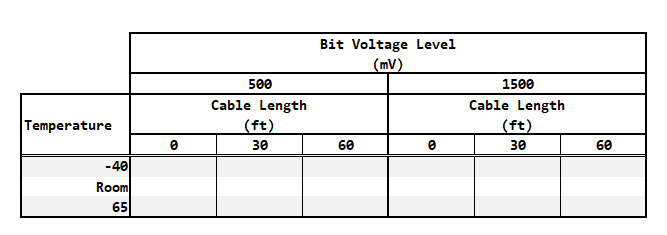# Test Time vs BER and Confidence Level Using Excel

Quote of the Day

When a thing is done, it's done. Don't look back. Look forward to your next objective.

— George C. Marshall. I have met many people who spend their lives constantly looking back and expressing regret for opportunities lost. Learn what you can from your experience and move forward. As Spock used to say "Regret is a useless emotion, especially in humans."Figure 1: BER Test Data Table for a Single Unit. There are 18 measurements for each unit.

I am currently working as a project manager on a serial data channel qualification task. During this work, I need to estimate the time required to perform dozens of Bit Error Rate (BER) measurements under different scenarios (see Figure 1). In these measurements, we are working to ensure that our BER is always 1E-10 or less. I have performed this calculation many times and have derived all the associated formulas here. BER is a statistical parameter and its measurement is a function of the confidence level you want in your answer.  In this case, we want the highest confidence level possible consistent with a two-week test effort.

The calculation requires solving Equation 1 for n, the number of bits required, to confirm a maximum BER p at a specific confidence level CL and error number N.

 Eq. 1$-n\cdot p=\ln \left( 1-CL \right)-\ln \left( \sum\limits_{k=0}^{N}{\frac{{{\left( np \right)}^{k}}}{k!}} \right)$

To convert the number of bits transferred to a test time τ, we need to evaluate Equation 2 with the bit transfer rate, r.

 Eq. 2$\displaystyle \tau =\frac{n}{r}$

I normally perform this calculation using Mathcad, but in this case, other engineers wanted it written in Excel because they are familiar with that tool. The calculation is a bit messier in Excel because:

Figure 3 is a screenshot of the workbook's test time calculation.  The worksheet is easy to use – just input your p, N, CL, and rate values (highlighted in yellow), then press iterate. This calculation tells me how long a single BER measurement will take. Remember that I need to perform dozens of these measurements, so even small reductions in the single-test time make a big difference to the overall test time.

For those interested, my Excel workbook is here. It includes a very simple macro (shown below) that calls the Goal Seek function for each confidence level and allowed error number. The macro also changes the iteration setting.

This entry was posted in Electronics, Excel. Bookmark the permalink.